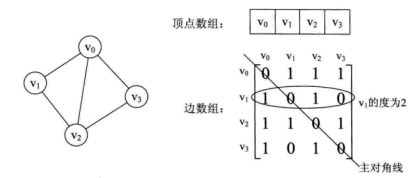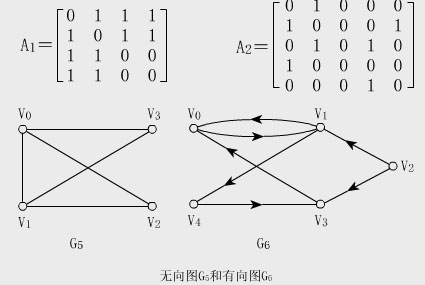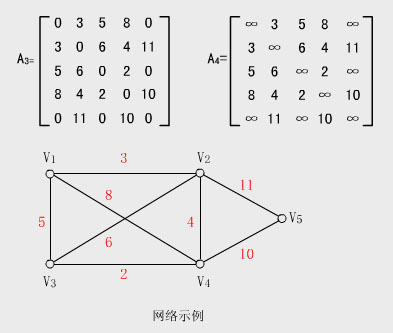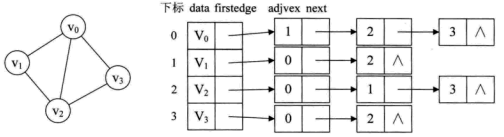• ## 邻接矩阵

万次阅读 2017-03-03 22:20:35
邻接矩阵分为有向图邻接矩阵和无向图邻接矩阵。 二，特点： 1),无向图的邻接矩阵一定是对称的，对于有n个顶点的无向图则只存上（下）三角阵中剔除了左上右下对角线上的0元素后剩余的元素，故只需1+2+.....+(n-1)=n*...
一，逻辑部分：
分为两部分：V和E集合。用一个一维数组存放所有顶点数据，用一个二维数组存放顶点间的关系数据，这个二维数组称为邻接矩阵。邻接矩阵分为有向图邻接矩阵和无向图邻接矩阵。
二，特点：
1),无向图的邻接矩阵一定是对称的，对于有n个顶点的无向图则只存上（下）三角阵中剔除了左上右下对角线上的0元素后剩余的元素，故只需1+2+.....+(n-1)=n*(n-1)/2个单元。
2），有向图的邻接矩阵不一定对称，表示图共需n^2个空间。
三，表示法：
1),用邻接矩阵表示顶点间的相邻关系
2)，用一个顺序表来存储顶点信息
图的矩阵
设G=(V,E)是具有n个顶点的图，则G的邻接矩阵是具有如下性质的n阶方阵：举例：

下图中
无向图G 5 和
有向图G 6 的邻接
矩阵分别为A1 和A 2 。网络矩阵：

若G是网络，则邻接
矩阵
可定义为：

w ij 表示边上的权值;

∞表示一个计算机允许的、大于所有边上权值的数。举例：下面带权图的两种邻接矩阵分别为A 3 和A 4 。四，代码示例：
#include<iostream> using namespace std; #define MAXVEX 100/* 最大顶点数，应由用户定义 */ #define INF 65535 /* 表示权值的无穷*/ //typedef int EdgeType;/* 边上的权值类型应由用户定义 */ //typedef char VertexType;/* 顶点类型应由用户定义  */ typedef struct {     char vexs[MAXVEX];/* 顶点表 */     int arc[MAXVEX][MAXVEX];/* 邻接矩阵，可看作边表 */     int numNodes, numEdges;/* 图中当前的顶点数和边数  */ } MGraph; /* 建立无向网图的邻接矩阵表示 */ void CreateMGraph(MGraph *Gp) {     int i, j, k, w;     cout << "请输入顶点数和边数（空格分隔）：" << endl;     cin >> Gp->numNodes >> Gp->numEdges;     cout << "请输入顶点信息（空格分隔）：" << endl;     for (i = 0; i < Gp->numNodes; i++)         cin >> Gp->vexs[i];     for (i = 0; i < Gp->numNodes; i++)     {         for (j = 0; j < Gp->numNodes; j++)         {             if (i == j)                 Gp->arc[i][j] = 0;/* 顶点没有到自己的边*/             else                 Gp->arc[i][j] = INF;/* 邻接矩阵初始化 */         }     }      for (k = 0; k < Gp->numEdges; k++)     {         cout << "请输入边（vi, vj)的上标i，下标j和权值w（空格分隔）:" << endl;         cin >> i >> j >> w;         Gp->arc[i][j] = w;         Gp->arc[j][i] = Gp->arc[i][j];/* 因为是无向图，矩阵对称 */     } }  int main(void) {     MGraph MG;     CreateMGraph(&MG);      return 0; }

展开全文• **关于图的实现方法，除了邻接矩阵还有邻接表等方法，会在后续博客中写出 邻接矩阵（即数组）来实现图难度不高，步骤分析如下： 第一步： 建立邻接矩阵 struct GNode{ int Nv;//图的顶点数 int Ne;//图的边数 ...
邻接矩阵（Adjacency Matrix）是表示顶点之间相邻关系的矩阵。设G=(V,E)是一个图，其中V={v1,v2,…,vn}  。G的邻接矩阵是一个具有下列性质的n阶方阵： ①对无向图而言，邻接矩阵一定是对称的，而且主对角线一定为零（在此仅讨论无向简单图），副对角线不一定为0，有向图则不一定如此。 ②在无向图中，任一顶点i的度为第i列（或第i行）所有非零元素的个数，在有向图中顶点i的出度为第i行所有非零元素的个数，而入度为第i列所有非零元素的个数。 ③用邻接矩阵法表示图共需要n^2个空间，由于无向图的邻接矩阵一定具有对称关系，所以扣除对角线为零外，仅需要存储上三角形或下三角形的数据即可，因此仅需要n（n-1）/2个空间。
关于图的实现方法，除了邻接矩阵还有邻接表等方法，会在后续博客中写出 邻接矩阵（即数组）来实现图难度不高，步骤分析如下：第一步： 建立邻接矩阵
struct GNode{
int Nv;//图的顶点数
int Ne;//图的边数
int G[MaxVertexNum][MaxVertexNum];//二维数组
};
typedef struct GNode *PtrToGNode;//此为指向GNode的指针
typedef PtrToGNode MGraph;//定义为MGraph

第二步： 初始化图
typedef int Vertex;
MGraph CreateGraph(int VertexNum){
Vertex v,w;
MGraph Graph;
Graph=(MGraph)malloc(sizeof(struct GNode));
Graph->Nv=VertexNum;
Graph->Ne=0;
//顶点默认从编号0开始
for(v=0;v<Graph->Nv;v++){
for(w=0;w<Graph->Nv;w++){
Graph->G[v][w]=0;
}
}
return Graph;
}

第三步： 定义边以及定义插入边函数
struct ENode{
Vertex v1,v2;//顶点v1，v2
int Weight;
};

typedef struct ENode *PtrToENode;
typedef PtrToENode Edge;

void InsertEdge(MGraph Graph,Edge E){
//插入边<v1,v2>
Graph->G[E->v1][E->v2]=E->Weight;
//若是无向图，则还要插入<v2,v1>
Graph->G[E->v2][E->v1]=E->Weight;
}

第四步： 完整的建立一个邻接矩阵图
MGraph BuildGraph(){
MGraph Graph;
Edge E;
Vertex v;
int Nv,i;
printf("请输入顶点数：");
scanf("%d",&Nv);
Graph=CreateGraph(Nv);
print("请输入边数：");
scanf("%d",&Graph->Ne);
if(Graph->Ne!=0){
E=(Edge)malloc(sizeof(struct ENode));
for(i=0;i<Graph->Ne;i++){
printf("请输入两个顶点与边的权重，用空格分隔输入\n");
scanf("%d%d%d",&E->v1,&E->v2,&E->Weight);
InsertEdge(Graph,E);
}
}

}

这样就完成了一个简单的邻接矩阵图的建立了。此处没有考虑顶点需要存储数据的情况，可自行添加代码完善噢。
														By Dewitt

**
展开全文数据结构 c语言 算法
• ## 邻接矩阵和邻接表

千次阅读 2020-07-05 19:30:41
图的存储结构主要分两种，一种是邻接矩阵，一种是邻接表。 1.邻接矩阵 邻接矩阵的存储方式是用两个数组来表示图。一个一维数组储存图中顶点信息，一个二维数组储存图中的边或弧的信息。 无向图 这里右图是把...
图的存储结构主要分两种，一种是邻接矩阵，一种是邻接表。
1.邻接矩阵
邻接矩阵的存储方式是用两个数组来表示图。一个一维数组储存图中顶点信息，一个二维数组储存图中的边或弧的信息。
无向图这里右图是把顶点作为矩阵内行和列的标头罗列出来。如果在两个顶点之间存在一条边，那么就把 1 放在这个位置上。如果边不存在，那么就赋值为 0。
从上面可以看出，无向图的边数组是一个对称矩阵。所谓对称矩阵就是n阶矩阵的元满足aij = aji。即从矩阵的左上角到右下角的主对角线为轴，右上角的元和左下角相对应的元全都是相等的。
从矩阵中很容易分析到图中信息：
1）判断顶点之间是否有边很容易；
2）要知道顶点的度，其实就是这个顶点在第i行(或第i列)的元素之和；
3）求顶点vi的所有邻接点就是将矩阵中第i行元素扫描一遍，arc[i][j]为1就是邻接点。
有向图上图图右是一个有向图。有向图是看入度和出度的(顶点的入边数和出边数)，例如V2，就有2个出边。列代表着顶点的入边，列的元素相加代表顶点的入度，而行则代表该顶点所有的出边，元素相加则为顶点的出度。假如V2->V1的这个关系要写到邻接矩阵，首先先找到V1的列，然后在对应V2行的位置写上一个1，就可以了。
网络
网络是一个带权图。邻接矩阵如下图：有两种表示方法，没有边的两个顶点，可以用0表示，也可以用最大值表示。
2.邻接表
邻接矩阵是不错的一种图存储结构，但是，对于边数相对顶点较少的图，这种结构存在对存储空间的极大浪费。因此，找到一种数组与链表相结合的存储方法称为邻接表。
邻接表的处理方法是这样的：        1）图中顶点用一个一维数组存储，当然，顶点也可以用单链表来存储，不过，数组可以较容易的读取顶点的信息，更加方便。        2）图中每个顶点vi的所有邻接点构成一个线性表，由于邻接点的个数不定，所以，用单链表存储，无向图称为顶点vi的边表，有向图则称为顶点vi作为弧尾的出边表(只管顶点的出边不管入边)。展开全文数据结构
问题描述 :
内容：

函数原型：
（1）void DFS_Traverse(int u); //DFS遍历（外壳部分，公有成员函数）
（2）bool DFS(int u, int &num, int visited[]); //DFS遍历（递归部分，私有成员函数）

辅助函数原型：
（1）int GetFirstAdjVex(int u, int &v); //返回G中指定顶点u的第一个邻接顶点的位序（顶点集）。若顶点在G中没有邻接顶点，则返回-1
（2）int GetNextAdjVex(int u, int v, int &w); //返回G中指定顶点u的下一个邻接顶点（相对于v）的位序（顶点集）。若顶点在G中没有邻接顶点，则返回-1

注意：DG（有向图）, DN（有向网）, UDG（无向图）, UDN（无向网）

图的邻接矩阵模板类原型参考如下：

template <class TypeOfVer, class TypeOfEdge>
private:
int Vers;        //顶点数
int Edges;       //边数
TypeOfEdge **edge;  //存放邻接矩阵（TypeOfEdge表示顶点关系类型。对于无权图，用1或0，表示相邻否；对于带权图，则为权值类型）
TypeOfVer *ver;    //存放结点值
TypeOfEdge noEdge;  //邻接矩阵中的∞的表示值
string GraphKind;   //图的种类标志

bool DFS(int u, int &num, int visited[]); //DFS遍历（递归部分）

public:
adjmatrix_graph( const string &kd, int vSize, const TypeOfVer d[], const TypeOfEdge noEdgeFlag); //构造函数构造一个只有结点没有边的图。4个参数的含义：图的类型、结点数、结点值和邻接矩阵中表示结点间没有边的标记（无权图：0，有权图：输入参数定）
adjmatrix_graph( const string &kd, int vSize, int eSize, const TypeOfVer d[], int **e); //构造函数构造一个无权图。5个参数的含义：图的类型、结点数、边数、结点集和边集
adjmatrix_graph( const string &kd, int vSize, int eSize, const TypeOfEdge noEdgeFlag, const TypeOfVer d[], int **e, const TypeOfEdge w[]); //构造函数构造一个有权图。7个参数的含义：图的类型、结点数、边数、无边标记、结点集、边集、权集
bool GraphisEmpty() { return Vers == 0; }  //判断图空否
string GetGraphKind(){ return GraphKind; }
bool GetVer(int u, TypeOfVer &data); //取得G中指定顶点的值
int GetFirstAdjVex(int u, int &v); //返回G中指定顶点u的第一个邻接顶点的位序（顶点集）。若顶点在G中没有邻接顶点，则返回-1
int GetNextAdjVex(int u, int v, int &w); //返回G中指定顶点u的下一个邻接顶点（相对于v）的位序（顶点集）。若顶点在G中没有邻接顶点，则返回-1
bool PutVer(int u, TypeOfVer data); //对G中指定顶点赋值
bool InsertVer(const TypeOfVer &data); //往G中添加一个顶点
int LocateVer(TypeOfVer data); //返回G中指定顶点的位置
bool PrintMatrix();  //输出邻接矩阵
int GetVerNum(){ return Vers;}    //取得当前顶点数
int GetEdgeNum(){ return Edges;}  //取得当前边数
bool Insert_Edge(int u, int v); //无权图插入一条边
bool Insert_Edge(int u, int v, TypeOfEdge w); //有权图插入一条边
bool DeleteVer(const TypeOfVer &data); //往G中删除一个顶点
bool Delete_Edge(int u, int v); //无权图删除一条边
bool Delete_Edge(int u, int v, TypeOfEdge w); //有权图删除一条边
void DFS_Traverse(int u); //DFS遍历（外壳部分）
void BFS_Traverse(int u); //BFS遍历
};

输入说明 :
建图的输入数据格式参见建图的算法说明。

第一行：图的类型
第二行：结点数
第三行：结点集
第四行：边数
第五行：边集
第六行：起始顶点的位序

输出说明 :
第一行：顶点集
空行
第二行：邻接矩阵
空行
第三行：DFS遍历序列（结点之间用->分隔）

输入范例 :

UDG 8 V1 V2 V3 V4 V5 V6 V7 V8 8 0 1 0 2 1 3 1 4 2 5 2 6 3 7 4 7 0

输出范例 :

V1 V2 V3 V4 V5 V6 V7 V8
0 1 1 0 0 0 0 0  1 0 0 1 1 0 0 0  1 0 0 0 0 1 1 0  0 1 0 0 0 0 0 1  0 1 0 0 0 0 0 1  0 0 1 0 0 0 0 0  0 0 1 0 0 0 0 0  0 0 0 1 1 0 0 0
V1->V2->V4->V8->V5->V3->V6->V7

解题代码：
#include <iostream>
#include <cstdio>
#include <cstdlib>
#include <algorithm>
#include <cmath>
#include <cstring>
#include <string>
#include <vector>
#include <queue>
#include <sstream>
#include <stack>
#include <map>
#include <ctime>
#include <array>
#include <set>
using namespace std;

template <class TypeOfVer, class TypeOfEdge>//节点数值，边数值
private:
int Vers;        //顶点(节点)数
int Edges;       //边数
//存放邻接矩阵（TypeOfEdge表示顶点关系类型。对于无权图，用1或0，表示相邻否；对于带权图，则为权值类型）

vector<vector<TypeOfEdge> >edge;//邻接矩阵
TypeOfEdge noEdge;  //邻接矩阵中的∞的表示值

vector<TypeOfVer> ver;    //存放结点值

string GraphKind;   //图的种类标志

bool have_dir = false, have_w = false;//图类型参数

vector<TypeOfVer> dfs_lis;
vector<bool> vis_lis;
bool DFS(int t) //DFS遍历（递归部分）
{
int i, j;
vis_lis[t] = true;
dfs_lis.push_back(ver[t]);
for (i = 0; i < Vers; i++)
if (edge[t][i] != noEdge)
if (vis_lis[i] == false)
DFS(i);
return 1;
}
public:
{
Vers = 0;
Edges = 0;
edge.clear();
ver.clear();
noEdge = 0;
}
{
;
}
//全自动输入 true is need 无边标记
bool Auto_input(bool need_emp)//true is need 无边标记
{
//DG（有向图）, DN（有向网）, UDG（无向图）, UDN（无向网）
/*第一行：图的类型  DN UDN
第二行：结点数
第三行：结点集
第四行：无边标记
第五行：边数
第六行：边集
第七行：权集*/

/*第一行：图的类型  DG UDG
第二行：结点数
第三行：结点集
第四行：边数
第五行：边集*/
cin >> GraphKind;//图的类型
cin >> Vers;//结点数
ver.resize(Vers);
for (int i = 0; i < Vers; i++)//结点集
cin >> ver[i];

if (need_emp)
cin >> noEdge;//无边标记

vector<TypeOfEdge> line;//邻接矩阵初始化
for (int j = 0; j < Vers; j++)
{
for (int i = 0; i < Vers; i++)
line.push_back(noEdge);
edge.push_back(line);
}

cin >> Edges;//边数
vector<int> x_p, y_p, w_p;
for (int i = 0; i < Edges; i++)
{
int c_x, c_y;
cin >> c_x >> c_y;
x_p.push_back(c_x);
y_p.push_back(c_y);
}
//图的类型识别

if (GraphKind == "DG")//DG（有向图）
have_dir = true, have_w = false;
if (GraphKind == "DN")//DN（有向网）
have_dir = true, have_w = true;
if (GraphKind == "UDG")//UDG（无向图）
have_dir = false, have_w = false;
if (GraphKind == "UDN")//UDN（无向网）
have_dir = false, have_w = true;

if(have_w)
for (int i = 0; i < Edges; i++)
{
int c_w;
cin >> c_w;
w_p.push_back(c_w);
}

for (int i = 0; i < Edges; i++)
{
if (have_dir == false)//无向图操作
{
if (have_w == true)//带权值的网的操作
edge[x_p[i]][y_p[i]] = edge[y_p[i]][x_p[i]] = w_p[i];
else//无权值操作
edge[x_p[i]][y_p[i]] = edge[y_p[i]][x_p[i]] = 1;
}
else
{
if (have_w == true)//带权值的网的操作
edge[x_p[i]][y_p[i]] = w_p[i];
else//无权值操作
edge[x_p[i]][y_p[i]] = 1;
}
}
return 1;
}
//输出邻接矩阵
bool PrintMatrix()
{
int i, j;
for (i = 0; i < Vers; i++)
{
for (j = 0; j < Vers-1; j++)
cout << edge[i][j] << " ";
cout << edge[i][j]<<" ";
cout << endl;
}
return 1;
}
//判断图空否
bool GraphisEmpty(void)
{
return Vers == 0;
}
//图的类型
string GetGraphKind(void)
{
return GraphKind;
}
//获得顶点集
vector<TypeOfVer> GetGraph_Point(void)
{
return ver;
}
//往G中添加一个顶点
bool InsertVer(const TypeOfVer& data)
{
ver.push_back(data);
vector<TypeOfEdge> line;//邻接矩阵一行
for (int j = 0; j < Vers; j++)
{
edge[j].push_back(noEdge);
}
for (int j = 0; j <= Vers; j++)
{
line.push_back(noEdge);
}
edge.push_back(line);
Vers++;
return 1;
}
//返回G中指定顶点的位置
int LocateVer(TypeOfVer data)
{
int i = 0;
for (i = 0; i < ver.size(); i++)
if (ver[i] == data)
return i;
return -1;
}
//删除一个顶点
bool Delete_Point(const TypeOfVer& data)
{
int i, j;
for (i = 0; i < Vers; i++)
if (ver[i] == data)
{
ver.erase(ver.begin() + i);
break;
}
if (i == Vers)//没有找到
return 0;
Vers--;
edge.erase(edge.begin() + i);
for (j = 0; j < Vers; j++)
edge[j].erase(edge[j].begin() + i);
return 1;
}
//取得当前边数
int GetEdgeNum(void)
{
return Edges;
}
//取得当前顶点数
int GetVerNum()
{
return Vers;
}
//图删除一条边
bool Delete_Edge(int u, int v)
{
if (have_dir == true)
if (edge[u][v] != noEdge)
{
edge[u][v] = noEdge;
Edges--;
return true;
}
else
return false;
else
{
if (edge[u][v] != noEdge && edge[v][u] != noEdge)
{
edge[u][v] = noEdge;
edge[v][u] = noEdge;
Edges--;
return true;
}
else
return false;
}
return false;
}
//取得G中指定顶点的入度
int search_PointIn(int p)
{
if (have_dir == false)//无向图无入度
return -1;
if (p < 0 || p >= Vers)
return -1;
int i,ans=0;
for (i = 0; i < Vers; i++)
if (edge[i][p] != noEdge)
ans++;
return ans;
}
int search_PointOut(int p)//取得G中指定顶点的出度
{
if (p < 0 || p >= Vers)
return -1;
int i, ans = 0;
for (i = 0; i < Vers; i++)
if (edge[p][i] != noEdge)
ans++;
return ans;
}
{
if (u < 0 || u >= Vers)
return false;
if (v < 0 || v >= Vers)
return false;
if (u == v)
return false;
if (edge[u][v] != noEdge)
return true;
else
return false;
}
int Look_firstPoint(int p)//获取第一个临界顶点位序
{
if (p < 0 || p >= Vers)
return -1;
for (int i = 0; i < Vers; i++)
if (edge[p][i] != noEdge)
return i;
return -1;
}
int Look_nextPoint(int u, int v)
{
if (u < 0 || u >= Vers)
return -1;
if (v < 0 || v >= Vers)
return -1;
for (int i = v+1; i < Vers; i++)
if (edge[u][i] != noEdge)
return i;
return -1;
}
vector<TypeOfVer> DFS_Traverse(int star)//深度优先遍历外壳
{
dfs_lis.clear();
vis_lis.clear();
for (int i = 0; i < Vers; i++)
vis_lis.push_back(false);
DFS(star);
return dfs_lis;
}
//+++++++++++++++++++======分割线=====+++++++++++++++++++++++++++++
//返回G中指定顶点u的第一个邻接顶点的位序（顶点集）。若顶点在G中没有邻接顶点，则返回-1
{
int p;
return p;
}
//返回G中指定顶点u的下一个邻接顶点（相对于v）的位序（顶点集）。若顶点在G中没有邻接顶点，则返回-1
int GetNextAdjVex(int u, int v, int& w)
{
return -1;
}
//对G中指定顶点赋值
bool PutVer(int u, TypeOfVer data)
{
return 1;
}

//无权图插入一条边
bool Insert_Edge(int u, int v)
{
return 1;
}
//有权图插入一条边
bool Insert_Edge(int u, int v, TypeOfEdge w)
{

return 1;
}

// //DFS遍历（外壳部分）

//void BFS_Traverse(int u); //BFS遍历

};
int main()
{
int i;
a.Auto_input(0);
int p;
cin >> p;
//cout << a.GetGraphKind() << endl;//输出类型
//输出顶点集----------------------
vector<string> ans;
ans = a.GetGraph_Point();
for (i = 0; i < ans.size() - 1; i++)
cout << ans[i] << " ";
cout << ans[i] << endl;
//-------------------------------
cout << endl;
a.PrintMatrix();
cout << endl;

vector<string> dfs_list;
dfs_list = a.DFS_Traverse(p);
for (i = 0; i < dfs_list.size() - 1; i++)
cout << dfs_list[i] << "->";
cout << dfs_list[i] << endl;
return 0;
}


展开全文• ## 邻接表和邻接矩阵

千次阅读 2018-09-19 16:20:31
进入图论的大门(深渊)之前,不会存图可不行,来来来,邻接表和邻接矩阵拿去花
• 目录 邻接矩阵 百度百科的定义 ...好了，今天想介绍的是邻接矩阵以及邻接表（先一点，之后补充） 先放一张邻接表和邻接矩阵对比： 邻接矩阵 ————————————同济第六版线性代数.
• 邻接矩阵以及邻接表(彻底搞懂到自己会用) 邻接矩阵： f[i][j]//表示i到j有没有边,以及是否关联，存入权值 关联矩阵： n行e列 f[i][j]//若有边，存入1,否则存入0 实例： → 当边数很稀疏时（m<<n^2）用相邻...
• 邻接矩阵 关联矩阵 对于图G=(V,E), 点数为n，边数为m； 1. 邻接矩阵A 1.1 定义 行为顶点，列也为顶点 的n*n矩阵。矩阵元素aij=vi与vj之间关联的边数。 若vi与vj不邻接，则aij=0. 1.2 性质 A是非负的、...关联矩阵
• 邻接矩阵 邻接矩阵（Adjacency Matrix）是表示顶点之间相邻关系的矩阵。设G=(V,E)是一个图，其中V={v1,v2,…,vn}  。G的邻接矩阵是一个具有下列性质的n阶方阵： ①对无向图而言，邻接矩阵一定是对称的，而且主对...数据结构
• 图的C++的邻接表和邻接矩阵完整实现，功能齐全，运行界面效果好代码
• ## 数据结构 图的邻接矩阵

万次阅读 多人点赞 2018-05-07 21:19:59
图的邻接矩阵的存储方式是用两个数组来实现的，一个一维数组存储顶点信息，一个二维数组存储线（无向图）或弧（有向图）的信息。设图G有n个顶点，则邻接矩阵是一个n × n的方阵，定义为：无向图的邻接矩阵，两个顶点...
• 掌握邻接矩阵和邻接链表建立图的存储结构；掌握DFS、BFS的基本思想及对图的遍历操作；了解图结构的应用。 二、实验内容： 设计一个有向图和一个无向图，使用邻接矩阵和邻接表存储结构，完成在这两种存储结构下有向图...
•邻接表 广度优先搜索 深度优先搜索
• ## 邻接矩阵与关联矩阵

万次阅读 多人点赞 2017-02-18 19:53:03
邻接矩阵】 定义： 设无向图G=(V,E)G=(V,E)G=(V,E),其中顶点集V=v1,v2,...,vnV=v1,v2,...,vnV={v_1,v_2,...,v_n}，边集E=e1,e2,...,eεE=e1,e2,...,eεE={e_1,e_2,...,e_\varepsilon}。用aijaija_{ij}表示顶点...
• ## 图的邻接矩阵（C语言）

万次阅读 多人点赞 2017-08-18 17:57:34
邻接矩阵 无向图和有向图在邻接矩阵中的表示方法： 无向图和有向图大同小异，在这里只以无向图为例，代码部分通过简单调整即可对应编译有向图邻接矩阵数据类型定义#define MaxVertices 100 //定义最大容量 typedef...c语言 数据
• 1、如何根据有向图画出邻接矩阵？ 如图： v1指向v2和v3，在矩阵中v1指向v2、v3的表示标1。 注意： v1指向v2在矩阵中是用竖列的v1对应横行的v2   2、如何根据有向图画出邻接表呢？ 注意： 画有向图的邻接表...
• 图的邻接矩阵和邻接表 转自：https://www.cnblogs.com/luoyang0515/p/10843932.html 许多人到这一块会比较混乱，特别是邻接表，定义的东西很多，同时也为自己做一个总结。 打算以图的深度优先搜索为例，分别表示邻接...邻接表 深度优先搜索 无向图
• ## 邻接矩阵无向图

千次阅读 2017-12-29 21:15:58
邻接矩阵   无向图和有向图在邻接矩阵中的表示方法：  无向图和有向图大同小异，在这里只以无向图为例，代码部分通过简单调整即可对应编译有向图 邻接矩阵数据类型定义 #define MaxVertices 100 //...
• 出入度 邻接表邻接矩阵 拓扑排序 DFS Kahn算法出入度一般指的是在有向图（DAG）中，某个顶点，箭头指向它的为入度...邻接表、邻接矩阵邻接表这个东西也可以看【1】中是怎么这个邻接矩阵的，我更推荐的是看【2】中鱼c邻接表 拓扑排序
• 图有两种表示方法，邻接矩阵和邻接表，接下来我们讲解邻接矩阵和用ｃ实现一个邻接矩阵． 我们先看一个图： 我们想将这样一个图信息存储起来，我们有两个必须存储的数据，节点信息(a,b,c,d,e)和权值(3,5,4,1,6,7)...
• 邻接矩阵和邻接表： 示例图： 邻接矩阵 实现图的最简单的方法之一是使用二维矩阵。在该矩阵实现中，每个行和列表示图中的顶点。存储在行 v 和列 w 的交叉点处的单元中的值表示是否存在从顶点 v 到顶点 w 的边。...
• 图的邻接表表示转换成邻接矩阵表示的算法。 下面这个是有向图邻接表表示转换成邻接矩阵 #include #include #include int a;//邻接矩阵的载体 typedef struct ArcNode{ int adjvex; struct ...数据结构 邻接表
• 图的邻接矩阵表示法是用 两个数组 来表示 图的数据结构。一个是顶点数组，另一个是邻接矩阵数组。邻接矩阵 里存放着 顶点的关系。 用邻接矩阵表示图，在 看 顶点之间 是否有边，或者 求顶点的度等操作时比较简单。...图的数组表示法
• 之前的那份是用邻接矩阵访问的，最近在复习数据结构，决定把邻接表的也上来 邻接矩阵的看这里 : http://blog.csdn.net/hhooong/article/details/41761621 邻接表 ：(关键部分的算法)  void DFS ...
• 先初始化邻接矩阵。依次遍历各个顶点的边表，根据边表中记录的“改弧所指向的顶点的位置”修改邻接矩阵arc[i][j]的值。例如遍历第 i 行的时候（当前的顶点所在行数为 i ），依次遍历该顶点的边表结点，若当前顶点的...算法 数据结构 c++
• %% 无向图邻接矩阵和关联矩阵转换function w = incandadf(F,f)%F为输入无向图矩阵可以是邻接矩阵或关联矩阵%% 邻接矩阵转关联矩阵if f == 0 m = sum(sum(F))/2; n = size(F,1); w = zeros(n,m); k = 1; for i = 1:n ...
• 目录图的简介无向图（Graph）生成带权邻接矩阵求两点最短路径有向图（Digraph）生成带权邻接矩阵求最短路径 图的简介 图是拓扑学中的一个重要概念，分为无向图和有向图两种。图有两个重要属性，即点（Node）和边...Matlab 最短路径...